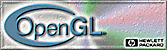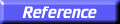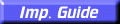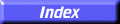# gluDisk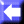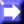gluDisk: draw a disk.

##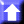C Specification

```void gluDisk(
GLdouble	 inner,
GLdouble	 outer,
GLint	 slices,
GLint	 loops)
```

##Parameters

inner
Specifies the inner radius of the disk (may be 0).
outer
Specifies the outer radius of the disk.
slices
Specifies the number of subdivisions around the z axis.
loops
Specifies the number of concentric rings about the origin into which the disk is subdivided.

##Description

gluDisk renders a disk on the z = 0 plane. The disk has a radius of outer, and contains a concentric circular hole with a radius of inner. If inner is 0, then no hole is generated. The disk is subdivided around the Z axis into slices (like pizza slices), and also about the Z axis into rings (as specified by slices and loops, respectively).

With respect to orientation, the +z side of the disk is considered to be "outside" (see gluQuadricOrientation). This means that if the orientation is set to GLU_OUTSIDE, then any normals generated point along the +Z axis. Otherwise, they point along the
Z axis.

If texturing has been turned on (with gluQuadricTexture), texture coordinates are generated linearly such that where r = outer, the value at (r, 0, 0) is (1, 0.5), at (0, r, 0) it is (0.5, 1), at (r, 0, 0) it is (0, 0.5), and at (0,r, 0) it is (0.5, 0).

gluCylinder,